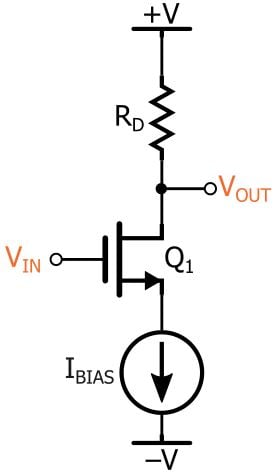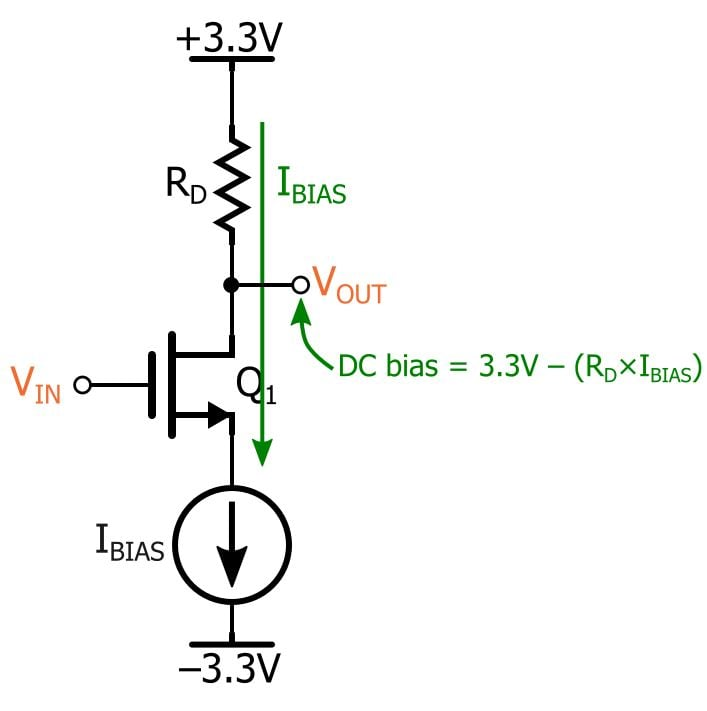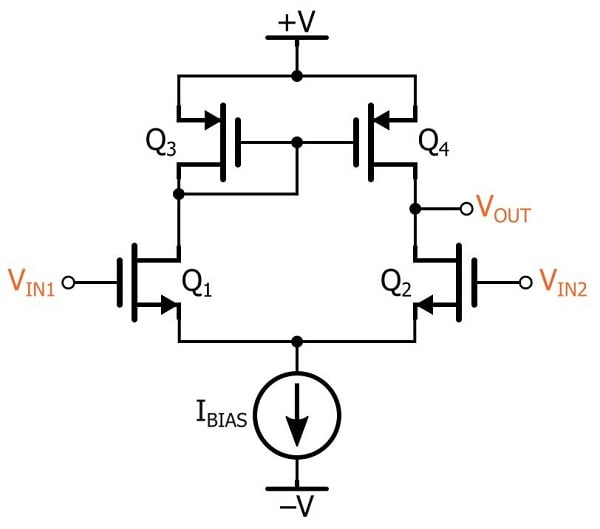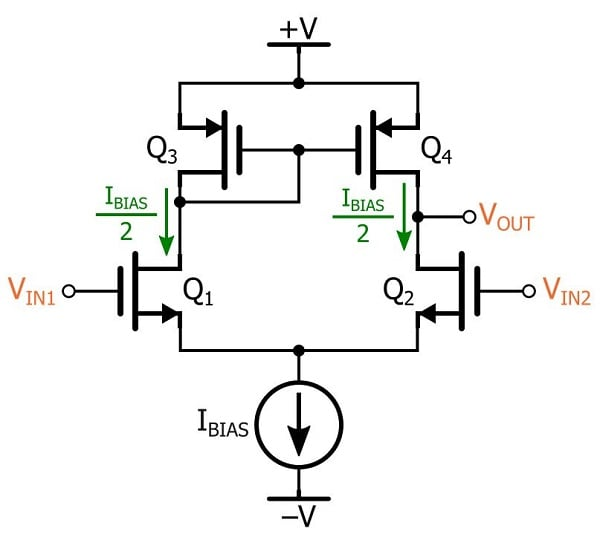Technical Article

# The MOSFET Differential Pair with Active Load

June 14, 2016 by Robert Keim

## Learn about a fairly simple yet highly beneficial modification to the drain-resistor-based version of the MOSFET differential pair.

Learn about a fairly simple yet highly beneficial modification to the drain-resistor-based version of the MOSFET differential pair.

### Supporting Information

Active loading is essential in the design of high-performance amplifiers. Though the circuitry involved is straightforward, the overall concept can be, in my opinion, somewhat abstruse. So we are going to take our time with this subject, with the primary goal (as usual) being a thorough, intuitive understanding.

Let’s start by looking at a passively loaded, non-differential MOSFET amplifier:The current source biases the FET so that it can operate in the saturation region. The overdrive voltage (VOV)—i.e., the gate-to-source voltage (VGS) minus the threshold voltage (VTH)—will be whatever value corresponds to a drain current of IBIAS. We are assuming that the input signal will be a small-amplitude sinusoid with no DC offset, and thus the source voltage will be equal to (0 – VOV).

What is the fundamental amplification mechanism in this circuit? Well, when biased in the saturation region, the FET acts like a voltage-controlled current source, with the drain current (if we ignore channel-length modulation) governed by the following equation:

$I_D=\frac{1}{2}\mu_nC_{ox}\frac{W}{L}(V_{GS}-V_{TH})^2$

Thus, our small-amplitude input signal will create small-amplitude variations in drain current. The ratio between small changes in input voltage and the resulting small changes in drain current is the transconductance, denoted by gm:

$\Delta I_D=\Delta V_{GS}\times g_m$

But the transconductance is not the same as the gain of the amplifier because we still need to convert this drain current into a voltage. This is the purpose of RD: It converts drain-current variations into drain-voltage variations. From Ohm’s law, we know that the ratio between the current variations and the voltage variations is the resistance RD, and thus the amplitude of the variations in drain voltage will be equal to the amplitude of the variations in gate voltage multiplied by gm multiplied by RD. If we recall that the gate is the input node and the drain is the output node, we can say that the magnitude of the voltage gain (AV) is the following:

$\frac{\Delta V_{OUT}}{\Delta V_{IN}}=A_V=g_m\times R_D$

So right away we see that one very easy way to increase the gain is to increase the value of the drain resistor. So why bother with the active load? If we want more gain, we just use more drain resistance!

### The Drain-Resistor Problem

There is one major problem with the more-drain-resistance approach: that resistance applies not only to small-signal variations in drain current but also to the larger steady-state drain current required for biasing. Consider the following diagram:So the drain resistor creates a biasing problem: More resistance means more voltage drop, and this means a lower bias-point voltage for the drain node. This may not seem like a serious concern if you’re thinking in terms of ±15 V supplies, but with ±3.3 V supplies, we need to be careful. If the drain voltage goes too low, the transistor will leave saturation and enter the triode region, and that’s not something we can tolerate—MOSFET amplifiers need to stay in saturation. Even if the bias voltage itself is not low enough to cause problems, too much negative signal swing could push the FET into the triode region. In either case, our amplifier is compromised.

So using large amounts of drain resistance is impractical, especially in modern low-voltage systems. How can we provide more small-signal resistance without introducing bias-point problems?

### Thinking About a Current Source

A resistor is a current-to-voltage converter. Remember that the unit for resistance, ohms, can be defined as volts per ampere: You put in I amps, you get out I × R volts.

Now let’s consider a current source in this same current-to-voltage-converter context. The current generated by an ideal current source never changes, even when the voltage across the terminals of the current source is extremely high. So, even the slightest change in current corresponds to an infinite change in voltage, and in this sense the current source is equivalent to an infinite resistance; this isn’t too surprising when we recall that DC network theorems require you to replace a current source with an open circuit.

The infinity thing can be distracting, so let’s transition now to a good-but-not-perfect current source. The equivalent resistance is very high, meaning that small changes in current lead to very large changes in voltage. If we could use this good-but-not-perfect current source instead of a drain resistor, we would have very high gain because small variations in gate voltage would produce corresponding variations in drain current and these, in turn, would produce large variations in drain voltage. Furthermore—and this is the critical point—the current source would not affect the bias conditions in the same way as the resistor because it’s a source of current, not just an obstacle to current.

The preceding discussion is rather abstract, but the concepts involved should help to sow the seed of understanding. Let’s transition now from the theoretical realm to the circuit realm.

### Two FETs and a Current Mirror

Here is the circuit:The bottom half of the differential pair is the same as what we would expect from the drain-resistor version. But now, instead of drain resistors, we have a PMOS current mirror. (We can see just by looking at the circuit that the gate voltage will be lower than the source voltage, and to get the FET out of cutoff with a negative gate-to-source voltage, we need PMOS.)

If you’ve read The Basic MOSFET Constant-Current Source (or if you’re otherwise familiar with current mirrors), you know that the “output” transistor (on the right) generates a relatively stable current that is proportional to the drain current of the “input” transistor (on the left), referred to as the reference current, IREF. The reference current, in turn, is determined by a resistor (i.e., the current-setting resistor, RSET).

So where is RSET for this mirror?

Well, in this case IREF is determined not by a resistor in the active-load mirror but by the IBIAS current source (which, in real life, would be a current mirror with a current-setting resistor). Also, if we assume perfect matching, the bias current will be divided equally between the two halves of the circuit (as with the drain-resistor-based differential pair). This means that the reference current is IBIAS/2. Thus, the input transistor of the active-load mirror has a reference current equal to IBIAS/2, and consequently the current generated by the output transistor will be IBIAS/2 (assuming that Q3 and Q4 have the same width-to-length ratio).### Conclusion

We’ll stop here for now. This article has provided a conceptual foundation, introduced the actively loaded differential pair, and presented the basic biasing conditions associated with the active-load current mirror. In the next article, we’ll continue our analysis of this important circuit.

Next Article in Series:

• Share• P
pa8821 January 09, 2018

In the first figure if the FET is biased by a current source, surely the drain current is fixed at exactly the current given by the current source. How can the FET act as a voltage controlled current source under these conditions?

Like.
•RK37 January 09, 2018
A fundamental aspect of analyzing transistor amplifiers is separating the large-signal conditions from the small-signal conditions. The current source establishes the large-signal (i.e., bias) conditions of the circuit, and the small-signal behavior (i.e., the amplifier behavior) is superimposed on the large-signal conditions. In the small-signal analysis for this circuit, the FET acts as a voltage-controlled current source, but this current doesn't "change" the value of the DC-biasing current source. Rather, it generates an output voltage by flowing to small-signal ground through the FET's small-signal output resistance in parallel with the drain resistance.
Like.
• B
bongo1234 June 22, 2018

•RK37 June 22, 2018